## Thresholding Techniques in Python using OpenCv

##### In this part of Learning Python we Cover Thresholding Techniques In Python
Written by |29-Apr-2019 | 0 Comments | 208 Views

In this lecture, we will continue the threshold. So now we will see how other thresholds work.

The threshold to Zero:

The threshold to zero manipulate the boundaries of a white pixel. Following is the code to implement this method:

`import cv2`
`import numpy as np`

`img = cv2.imread('doc.png',0)`
`_, thresh = cv2.threshold(img, 127, 255, cv2.THRESH_TOZERO)`
`cv2.imshow("img", thresh)`
`cv2.waitKey(0)`

The image generated is as follows: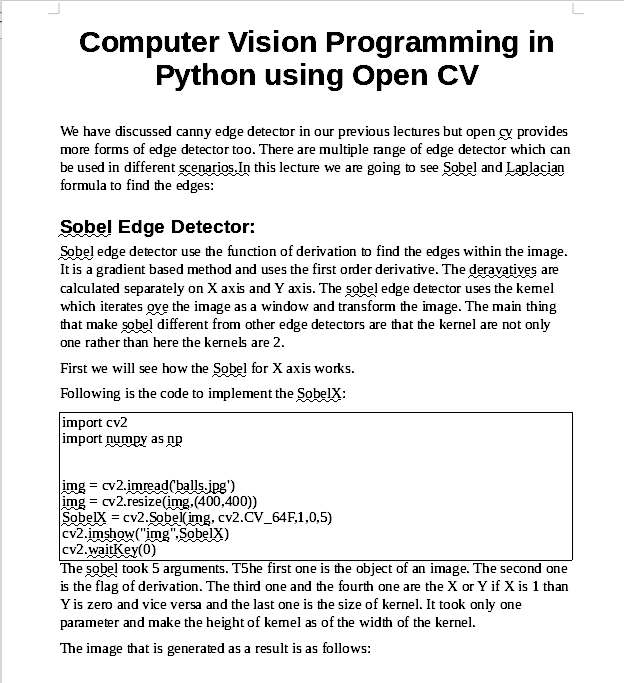Figure 1

The threshold to Zero Inverse:

It is the different method of the above method as it inverts the image. The thing that we can’t identify in the above Figure can be seen in this method. First, it will convert the black pixel to white and white to black, and then the words will be manipulated in an image. The code to implement this method is as follows:

`import cv2`
`import numpy as np`

`img = cv2.imread('doc.png',0)`
`_, thresh = cv2.threshold(img, 127, 255, cv2.THRESH_TOZERO_INV)`
`cv2.imshow("img", thresh)`
`cv2.waitKey(0)`

The image that is generated as follows: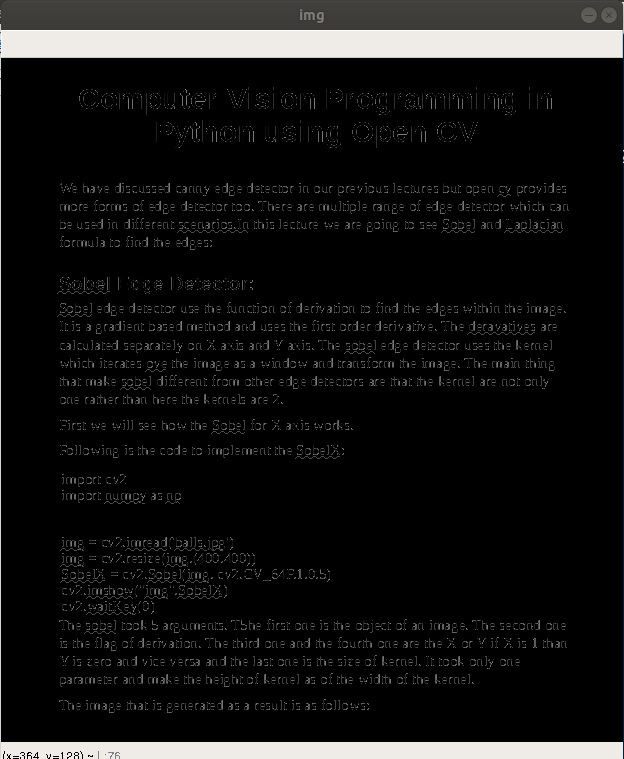Figure 2

Now we can see that the white pixels are not that clear; it becomes diminished. The letters written in bold have only their boundaries and are black in between.

In the previous methods, we have implemented the threshold that is applied to all part of the image which is not good at all. The different parts of an image can have different lighting effect and to use the same rule on all those parts cannot give the perfect results. So, to overcome this problem, there is another type of threshold known as the adaptive threshold. This threshold calculates the threshold for the small region of an image. It can vary from region to region which makes it more adaptive and more versatile.

Suppose we have an image of balls as follows: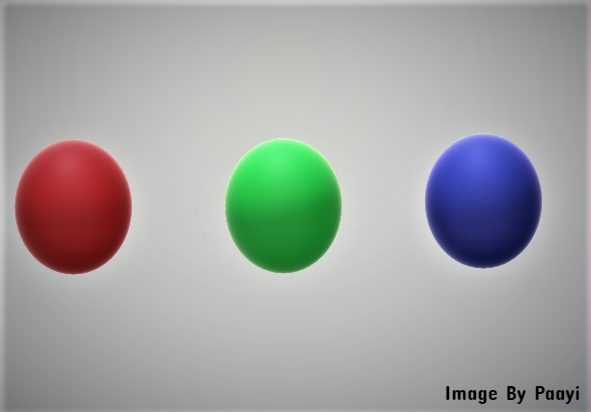Figure 3

The light intensity is different in different parts of an image. Let see what happen if we apply the reasonable threshold on this image as follows:

`import cv2`
`import numpy as np`

`img = cv2.imread('balls.jpg',0)`
`_,th = cv2.threshold(img,127,255,cv2.THRESH_BINARY)`

`cv2.imshow("img", th)`
`cv2.waitKey(0)`

The image generated as a result is as follows: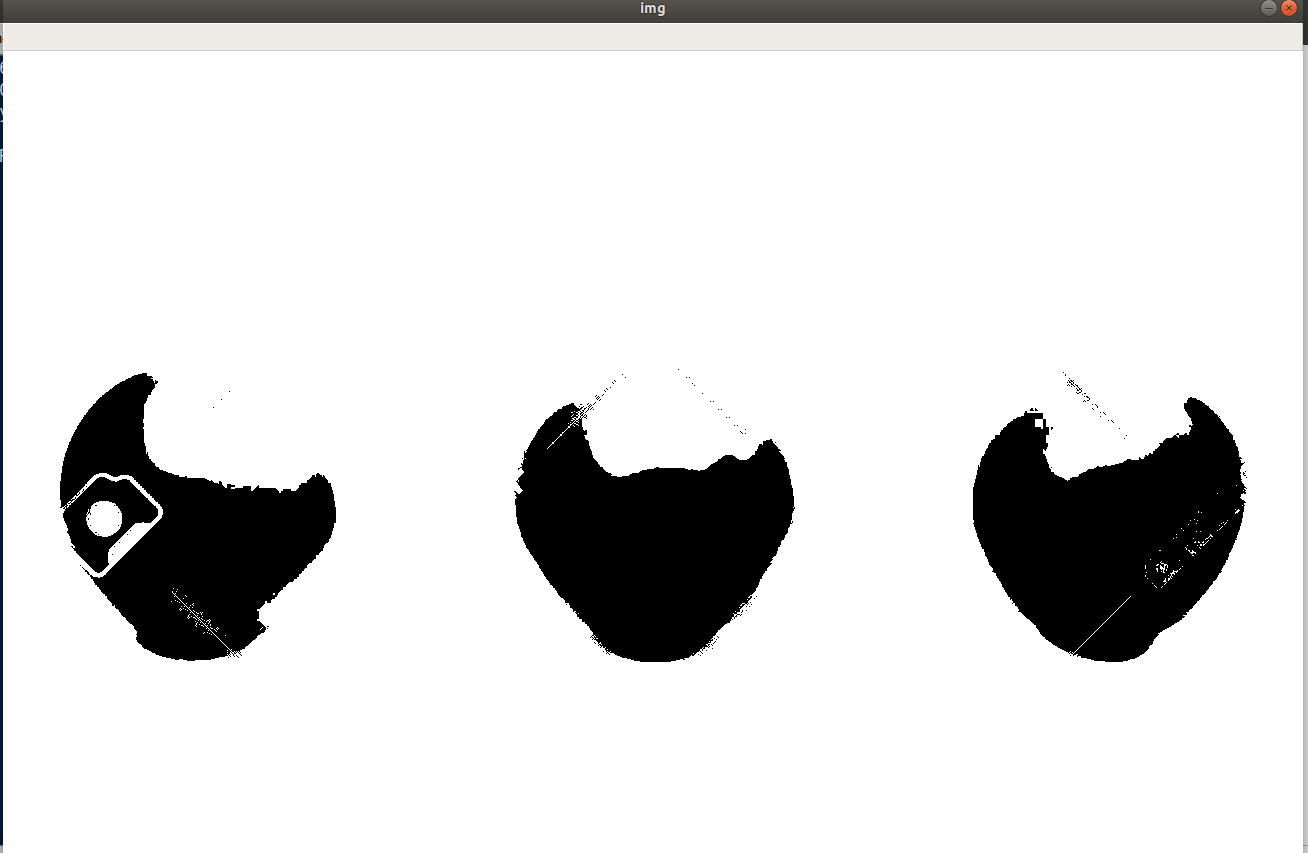Figure 4

We can see that it can’t identify the object and shape of the ball is also deformed. To tackle such a situation, we use the adaptive threshold. There are two types of adaptive threshold.

• Mean Threshold: The value of the threshold is the mean of the neighboring pixels
• Gaussian Threshold: The value of the threshold is the weighted sum of neighboring values.

In this method, the kernel iterates over the image and calculate the mean value. The threshold value depends on the mean of neighboring pixels. To demonstrate this method following method is to be implemented:

`import cv2`
`import numpy as np`

`img = cv2.imread('balls.jpg',0)`

`thresh = cv2.adaptiveThreshold(img, 255, cv2.ADAPTIVE_THRESH_MEAN_C, cv2.THRESH_BINARY, 11, 2)`
`cv2.imshow("img", th)`
`cv2.waitKey(0)`

The adaptive thresh takes six arguments. At first the object of an image. Similar to the above techniques it also took a grayscaled image. The second one is the maximum value. The third one, the flag which type of threshold we are going to use. In this case, we are using mean thresh, and there is another threshold that we used earlier. After that, we assign the block size. The block size is the kernel window which iterates over the image. Moreover, in last, there is a constant value which is subtracted from the mean or weighted sum value.

Now the image generated as a result from the above code is as follows: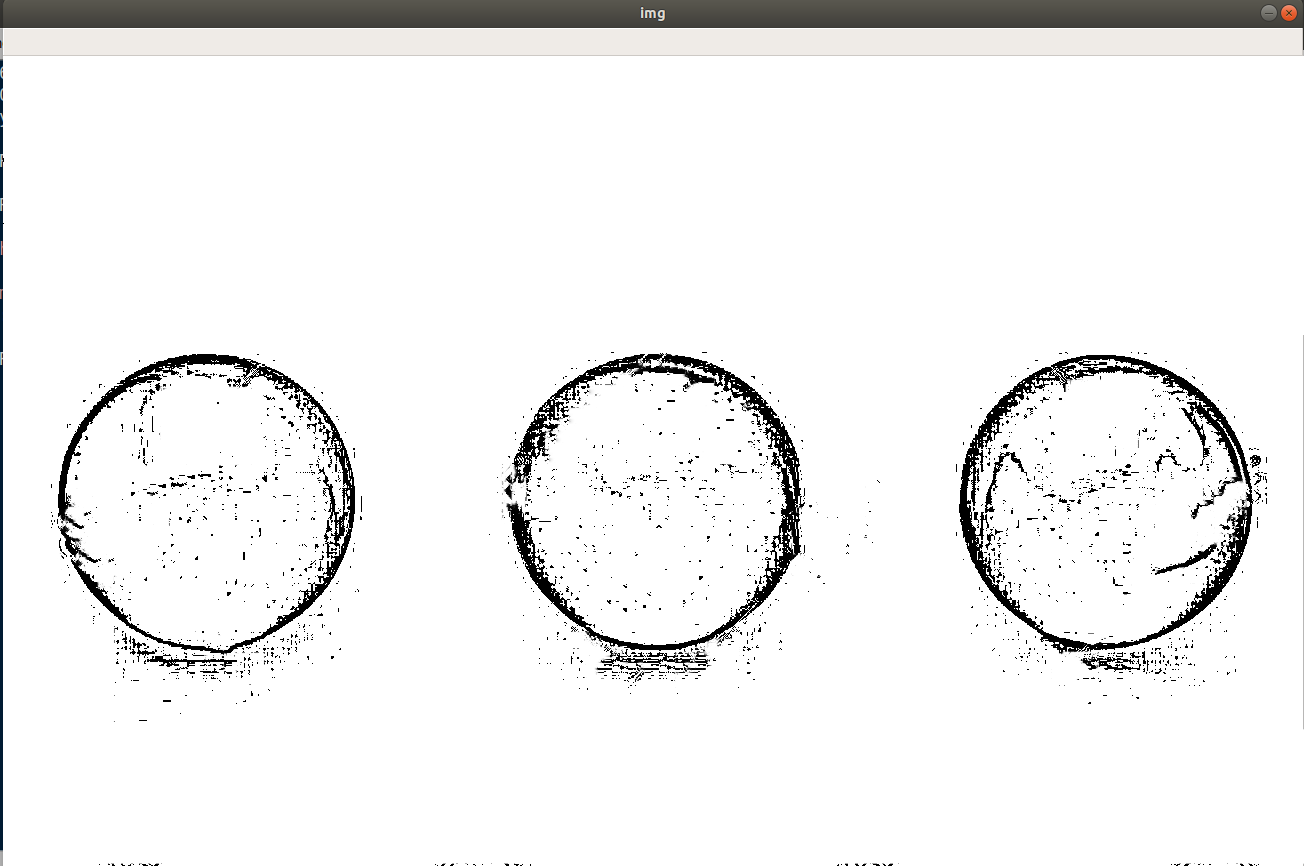Figure 5

Here we can see small details can be seen which was not possible with simple thresholding.

Gaussian Threshold:

In this method, kernel iterates over the image and calculate the weighted sum of all the pixels within the kernel window. The only difference between the code is the flag. Instead of using the flag of mean here we will use the flag of Gaussian.

Following is the code to implement the Gaussian threshold:

`import cv2`
`import numpy as np`

`img = cv2.imread('balls.jpg',0)`

`thresh = cv2.adaptiveThreshold(img, 255, cv2.ADAPTIVE_THRESH_GAUSSIAN_C, cv2.THRESH_BINARY, 11, 2)`
`cv2.imshow("img", thresh)`
`cv2.waitKey(0)`

The image that is generated as a result is as follows: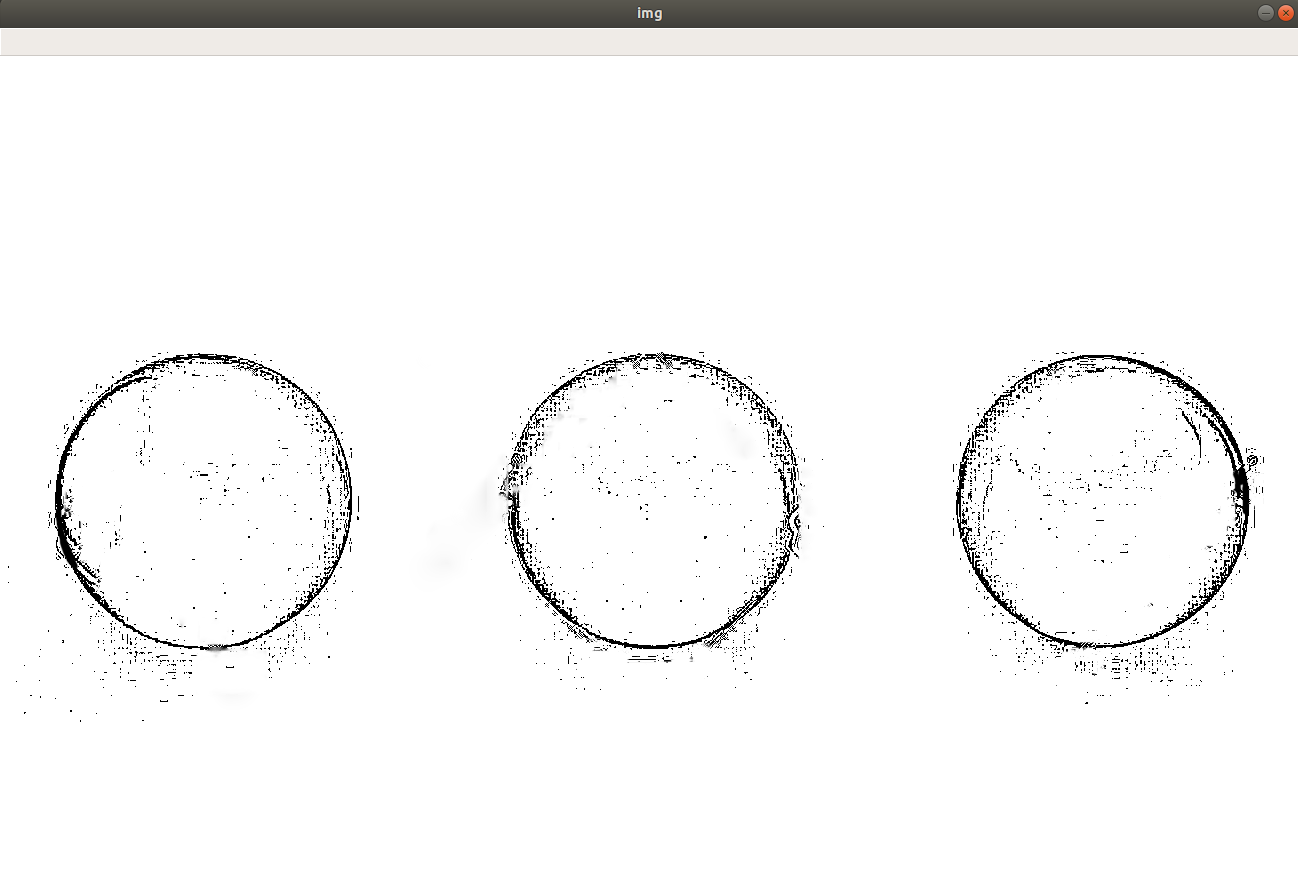Figure 6

We can see the slight difference between the two threshold images.

OTSU Binarization:

The OTSU binarization is another technique of getting the threshold of an image. So now the question arises what OTSU do? In simple words, the OTSU automatically calculate the threshold value of an image using the histogram of that image.

The Otsu is used with the simple threshold technique as follows:

`import cv2`
`import numpy as np`

`img = cv2.imread('balls.jpg',0)`
`_,th = cv2.threshold(img,127,255,cv2.THRESH_BINARY+cv2.THRESH_OTSU)`

`cv2.imshow("img", th)`
`cv2.waitKey(0)`

The image generated as a result is as follows: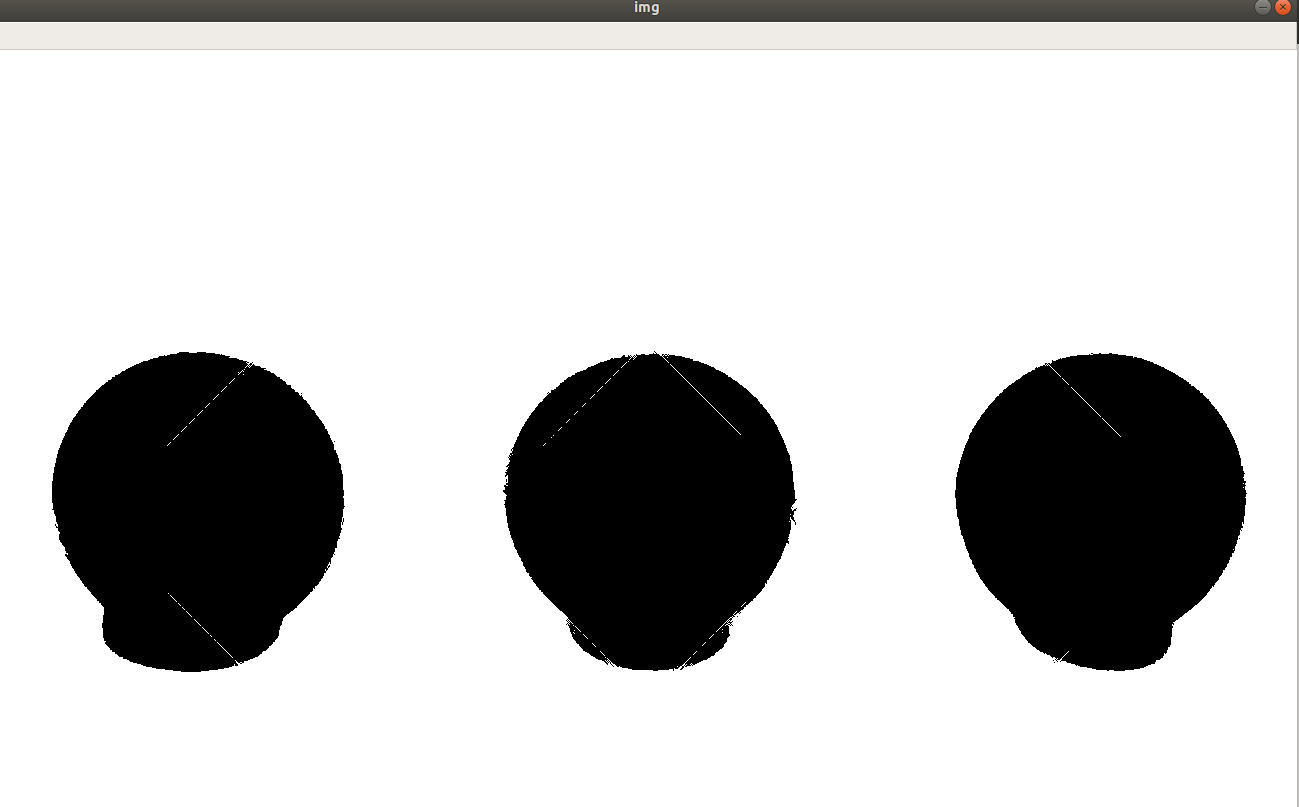Figure 7

Here we can see that the image is much clear that the previous all version of thresholding. So, we can say that the OTSU binary threshold is far most the excellent technique that we can use for thresholding.

Color Segmentation:

We have this image:Figure 8

Also, our task is to extract the green ball and minus all the colors from the image. This process is called the color segmentation method. To implement this following code is to be run:

`import cv2`
`import numpy as np`

`img = cv2.imread('balls.jpg')`
`hsv = cv2.cvtColor(img,cv2.COLOR_BGR2HSV)`
`lower = np.array([10,50,50])`
`upper = np.array([100,255,255])`
`mask = cv2.inRange(hsv,lower,upper)`
`res = cv2.bitwise_and(img,img,mask=mask)`
`cv2.imshow("img", res)`
`cv2.waitKey(0)`

At first, we read the image. After reading the image, we converted the image into HSV. HSV stands for Hue Saturated Value. The HSV image looks like the following: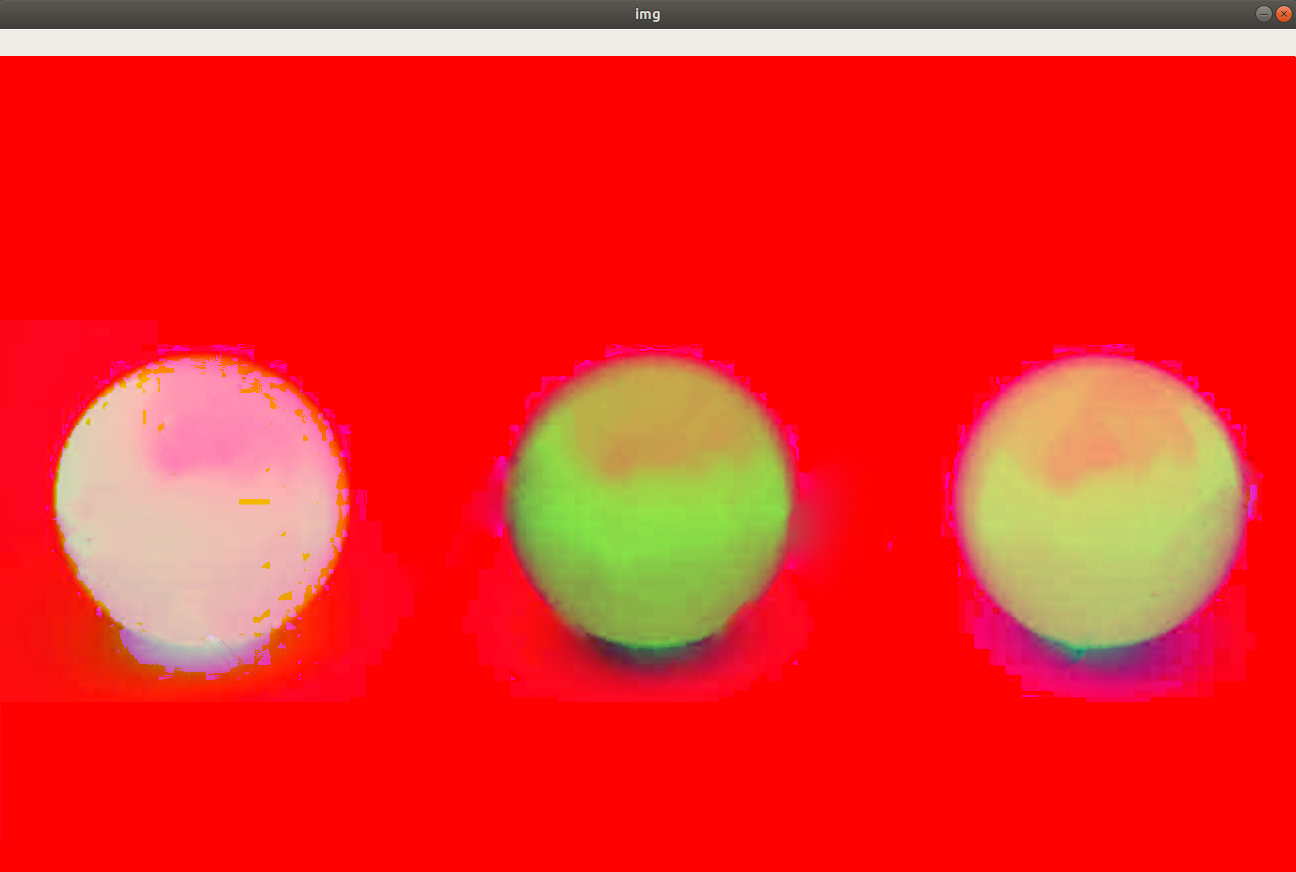Figure 9

It converts the image to red, blue and green color. After that, we make a range of upper and lower array to extract the green color you have to make changes if you want to detect different color. Then we create a mask and delete that mask from the frame which returns only the color we want. The result is as follows: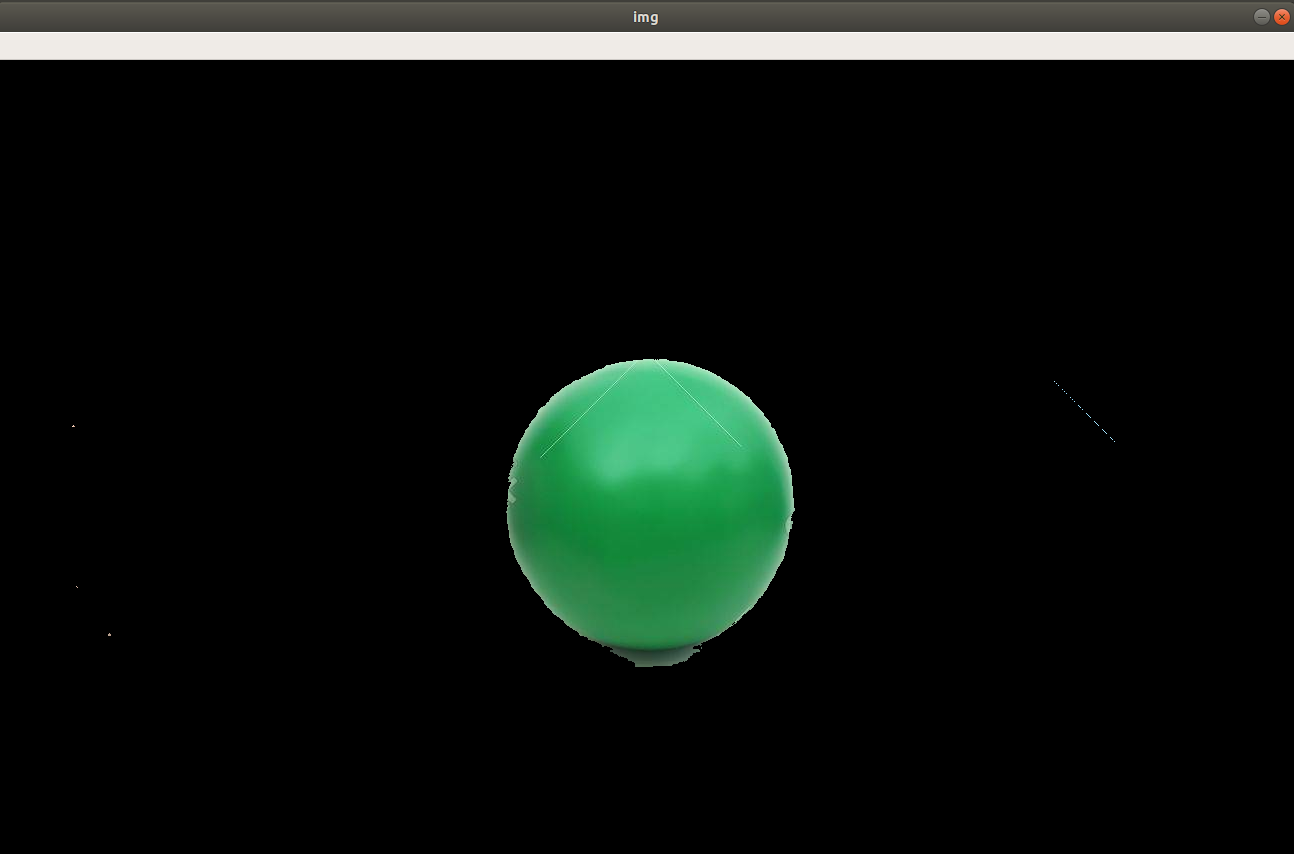Figure 10

As can be seen in Figure 10 that all other balls are deleted and only green is left.

Now we will continue the procedure to identify the red color in the image. By making the changes in lower and upper, we will make a change in the values of it. Following is the method to extract red color out of the image.

`import cv2`
`import numpy as np`

`img = cv2.imread('balls.jpg')`
`hsv = cv2.cvtColor(img,cv2.COLOR_BGR2HSV)`
`lower = np.array([170,100,100])`
`upper = np.array([200,255,255])`
`mask = cv2.inRange(hsv,lower,upper)`
`res = cv2.bitwise_and(img,img,mask=mask)`
`cv2.imshow("img", res)`
`cv2.waitKey(0)`

The code is the same as we have written earlier the only difference is that we have to change the values of lower and upper values which make it a different algorithm. The image that is generated as a result is as follows:Figure 11

In Figure 11 the green and blue balls are removed, and the only red ball is visible.